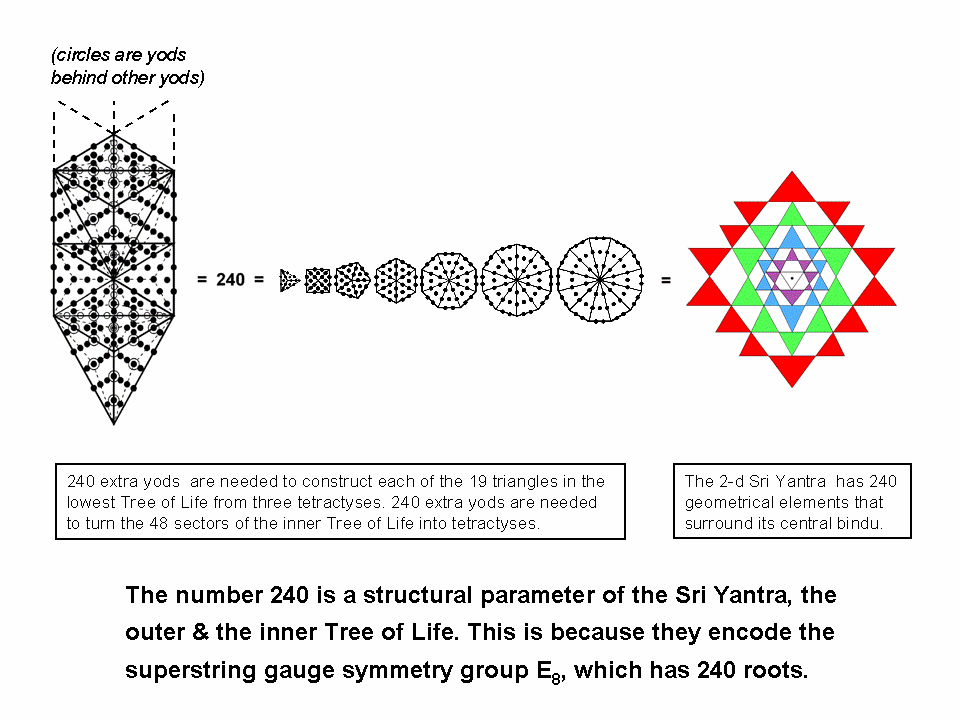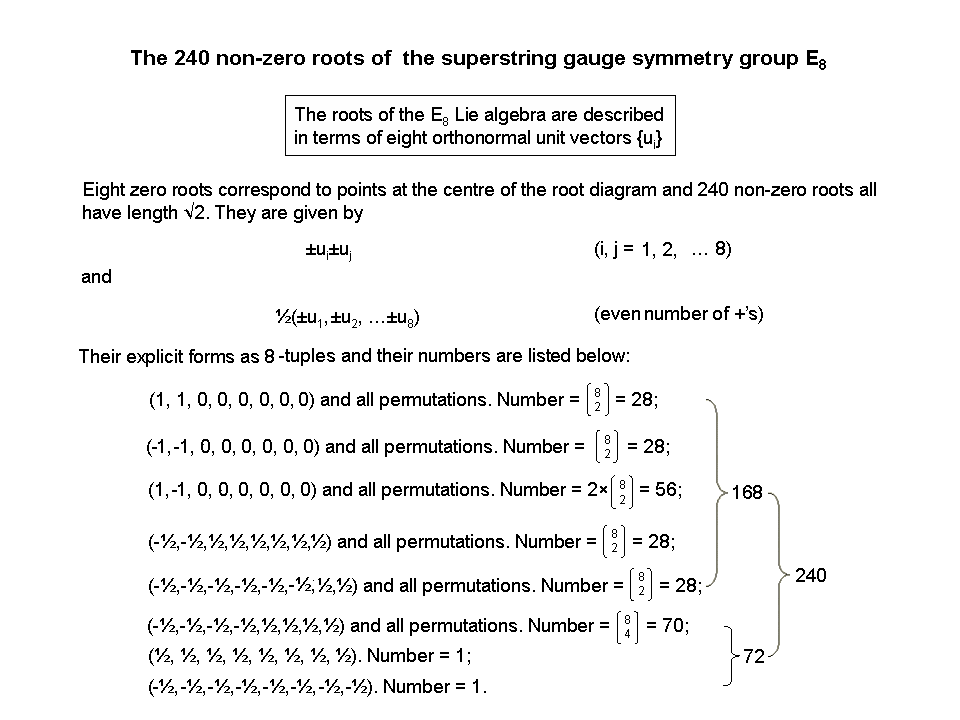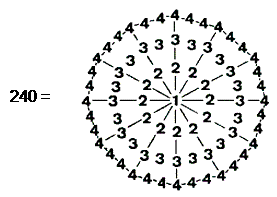<< Previous      2  3  4  5  ...56    Next >>

Sacred-geometrical basis of superstrings

#1 Sacred geometries embody the number 240 as the 240 non-zero roots of E8240 vertices & edges of the disdyakis triacontahedron surround an axis passing through two opposite vertices.The lowest Tree in any set of overlapping Trees of Life consists of 19 triangles. When their 57 sectors are turned into tetractyses, they contain 251 yods.* Therefore, starting with the 11 corners of these 19 triangles, the transformation of these triangles requires 240 more yods.

The inner Tree of Life consists of two sets of seven enfolded polygons. When separated and their 48 sectors turned into tetractyses, each set has 240 hexagonal yods, i.e., 240 more yods are needed to turn the sectors into tetractyses.

240 points, lines & triangles surround the centre of the 2-dimensional Sri Yantra. So, including its bindu, symbolising the Absolute behind the triple Godhead of Shiva, Vishnu & Brahma, the Sri Yantra comprises 241 geometrical elements. 241 is the 24th Pythagorean prime number,** where 24 = 4! = 1×2×3×4. This shows how the Tetrad determines arithmetically the geometrical composition of the 2-dimensional Sri Yantra.

The number 240 is the number of vertices (60) & edges (180) of the 120 triangular faces of the disdyakis triacontahedron that surround an axis passing through two diametrically opposite vertices. When its faces and the triangles inside it formed by its vertices and centre are divided into their sectors, 2400 (=240×10) corners, sides & triangular sectors surround an axis (see #1 here).

The number 240 is a defining parameter of all holistic systems possessing sacred geometry. It manifests in the E8×E8 heterotic superstring as the 240 gauge charges corresponding to the 240 non-zero roots of the rank-8, Lie group E8. They are represented by 240 vectors in an 8-dimensional vector space, their coordinates along each axis forming an 8-tuple (see also here). As explained in the following pages, these 240 gauge charges of E8 are distributed, 24 charges per whorl, in the ten whorls of the UPA — the subquark state of the E8×E8 heterotic superstring. This important conclusion is supported by the fact (proven in Article 53) that certain sacred-geometrical objects exhibit a 10-fold division of their geometrical or yod composition. The 10-fold nature of the UPA is the microscopic manifestation of this division:

 24 24 24 240 = 24 24 24 24 24 24 24

that is characteristic of sacred geometries.

When the Pythagorean integers 1, 2, 3 & 4 are assigned to the 73 yods in a Type A dodecagon, the sum of the 72 integers surrounding the central integer 1 is 240:The integer 1, which the ancient Pythagoreans regarded not as a number but as the source of all numbers, calling it the Monad, denotes the bindu at the centre of the Sri Yantra. This point symbolises the Absolute, the source of all things. The 72 Pythagorean integers surrounding it add up to the number of corners, sides & triangles in the 2-dimensional Sri Yantra that surrounding the bindu. The dodecagon is the tenth type of regular polygon, showing how the Decad determines the number of geometrical elements that surround the centre of the Sri Yantra. When the bindu is included, the Decad determines the number 241 in an arithmetic way because it is the 120th odd integer after 1, where 120 is the sum of the first ten odd integers after 1:

120 = 3 + 5 + 7 + 9 + 11 + 13 + 15 + 17 +19 + 21.

241 is the 121st odd integer, where 121 = 112 and 11 is the tenth integer after 1. This is another way in which the Decad can be seen to determine the number 240 as the measure of the geometrical composition of the 2-dimensional Sri Yantra. More generally, it explains why this number is a defining parameter of holistic systems, which always have a ten-fold pattern. Its role in the outer Tree of Life with 10 Sephiroth is obvious. Its mathematical influence on other sacred geometries is more subtle. The ten-fold UPA, the E8′-singlet state of the 10-dimensional E8×E8′ heterotic superstring, which carries the 240 gauge charges of E8, is par excellence the physical realization of the Decad.

* Proof: The 1-tree consists of 19 triangles with 11 corners and 25 sides. When each triangle is Type A, 10 yods are inside it. Number of yods in 1-tree with Type A triangles = 11 + 25×2 + 19×10 = 251.
** A Pythagorean prime number is of the form 4n + 1, where n is a positive integer.

 << Previous      2  3  4  5  ...56    Next >>

Home